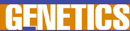# Mathematical Theories and Models of Genetics - Research Article from World of Genetics

This encyclopedia article consists of approximately 3 pages of information about Mathematical Theories and Models of Genetics.
 This section contains 743 words (approx. 3 pages at 300 words per page) View a FREE sampleMathematical theory and mathematical techniques are often critical tools in genetic research. Careful studies of data allow researchers to probe selected regions of molecules, or to characterize the distribution of genes in large populations. Incorporated into such studies are mathematical models for the genetic code and for molecular reactions (e.g., transcription and translation processes). In addition, mathematical models are used to study both the frequency and the mechanisms of genetic processes (e.g., mutation frequency or the number of possible errors in translation that can affect protein synthesis.

In addition to extensive use of statistical analysis, a number of other mathematical theories play an increasingly important role in genetics.

Group theory, for example, is one of several mathematical tools utilized to provide an accurate description of genetic molecules and processes. Of special interest to mathematicians and geneticists...

(read more)

 This section contains 743 words (approx. 3 pages at 300 words per page) View a FREE sampleCopyrightsMathematical Theories and Models of Genetics from Gale. ©2005-2006 Thomson Gale, a part of the Thomson Corporation. All rights reserved.